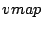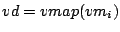Next: 5.5.0.0.1 Input: Up: 5 Towards an adaptive Previous: 5.4 VM assignment problems

## 5.5 Connected components

The simple online VM assignment problem can be formulated in terms of connected components. The connected components of a graph are a partitioning of the graph into subgraphs. A connected component of a directed graphis a maximal set of verticessuch that for every pair of verticesandin, there is a path connecting them. Hence two vertices are in the same connected component if and only if there exists a path between the vertices. If a graph contains only one connected component then it is said to be a connected graph.

The directed graphis a connected graph while the directed graphmay or may not be connected. It will be connected if and only ifthereat least onesuch that. Note that sinceis connected, the graph ofwheretheresuch thatsuch that, will also be connected.

Given an existing,, and their associated functions, we calculatewhereis a measure of the network cost value associated with each edge in the VM daemon graph. The cost value would be an integrated metric, a function of the measured available bandwidth,, and latency,, on the edges in the VM daemon graph.

The VM assignment problem may now be described as:

SubsectionsNext: 5.5.0.0.1 Input: Up: 5 Towards an adaptive Previous: 5.4 VM assignment problems
Ananth Sundararaj 2004-02-17